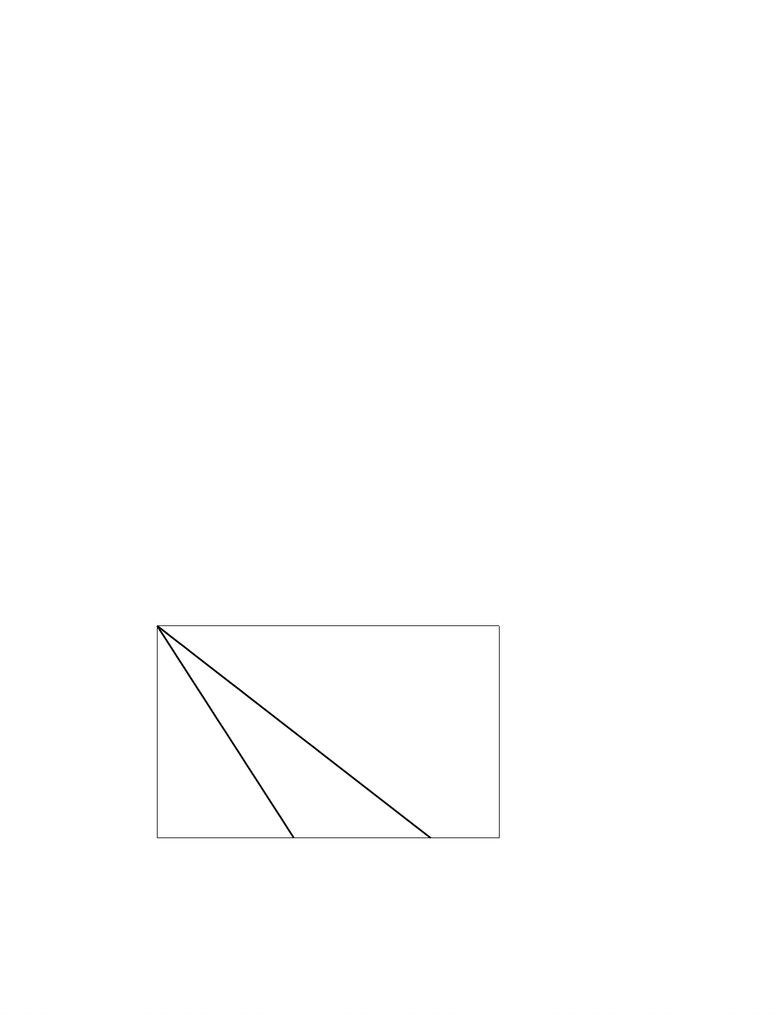# lec 5.doc

19 views10 pagesPrinciples of Economics: Monopoly
MONOPOLY
A monopoly (‘single sellers’) is the sole producer in an industry. This means that Demand
for the industry is Demand for the Monopoly and that the marginal cost and average cost
functions of the monopoly are the marginal cost and average cost functions for the industry. A
monopoly is not a price taker since a change in industry output changes the price of the
commodity for the monopoly. . Since price is not constant, marginal revenue is not equal to price
for a monopolist. The Marginal Revenue at a given output is always less than price at that
quantity since Marginal revenue (MR) = P + QP/Q (I derived this formula for marginal
revenue in an earlier lecture.) and P/Q < 0. (Marginal Revenue only equals Price for a
monopolist if market demand is perfectly elastic (horizontal), which is analogous to the perfect
elastic demand function facing the individual competitive firm) Since P is not constant and price
is dependent upon the output of the monopoly, a monopoly can determine commodity price by
restricting output. The marginal cost function of the monopoly is not the supply function for the
industry because quantity supplied is not simply determined by marginal cost in response to a
price but depends upon the the monopolist’s determination of price to maximize profit.
Profit Maximization for a Monopolist: MR = MC
Profit maximization for the monopolist occurs at the output where Marginal Cost =
Marginal Revenue (MC = MR) as we derived earlier for all firms. We cannot simplify this to P
= MC because MR ≠ P.
Deriving Marginal Revenue from Demand: SlopeMR = 2*SlopeD for Linear Demand
We know that Marginal Revenue = P + QP/Q but we can also express Marginal
Revenue in relation to the Demand function. In particular, Linear Demand functions generate
linear Marginal Revenues functions that have twice the slope of the Demand function.
- 1 -
Unlock document

This preview shows pages 1-3 of the document.
Unlock all 10 pages and 3 million more documents.Principles of Economics: Monopoly
Proof: A Linear Demand function is of the form P = a – bQ.
=> MR = TR/Q = (P*Q)/Q = (a – bQ)Q/Q = (a – bQ2)/Q = a – 2bQ
(MR = dTR/dQ = d(PQ)/dQ = d(a – bQ)Q/dQ = d(a – bQ2)/dQ = a – 2bQ)
i.e. Marginal Revenue for Linear Demand has the same intercept term as the Demand function
and twice the slope.
We can also say that quantity that gives a specific Marginal Revenue is half the quantity
from the Demand function that gives a Price equal to that Marginal Revenue.
Proof: If Demand is P = a – bQD so that Marginal Revenue is MR = a – 2bQMR, then
P = MR => a – bQD = a – 2bQMR, => bQD = 2bQMR
We already know this by the way from our understanding of the elasticity of a linear
Demand function. Elasticity is unit elastic at the midpoint of a Demand function, which means
that Marginal Revenue is 0 at the midpoint (bQD = 2QMR) because Total Revenue doesn’t change.
E.g. Suppose that Demand is P = 80 – 2Q. What is Marginal Revenue?
Marginal Revenue: MR = 80 – 4Q because slope of Marginal Revenue = 2(-2)
Demand: P = 80 - 2Q => MR = 80 - 4Q
0 10 20 30 40 50
0
20
40
60
80
P, MR
Q
Demand
MR
MR=0 => unit elasticity
- 2 -
Unlock document

This preview shows pages 1-3 of the document.
Unlock all 10 pages and 3 million more documents.Principles of Economics: Monopoly
A monopolist will not produce in the inelastic portion of a Demand function since a
decrease in quantity will a) increase Total Revenue and b) reduce Variable Costs. The
monopolist reduces quantity in the elastic portion of the Demand function so long as the
decreased Variable Cost of one less unit is greater than the decreased revenue from one less unit,
i.e., until MR = MC.
Monopoly Equilibrium and Economic Profit
Monopoly Equilibrium => monopoly output (QM) where MR = MC
=> Monopoly Price (PM) from Demand at QM
=> Average Cost (ACM)from Average Cost at QM
=> Economic Profit = (PM – ACM)*QM or PM*QM – Total CostM
E.g. Suppose that P = 80 – 2Q and Total Cost = Q2/2 + 5Q + 200 are the Demand and Total Cost
functions of an Industry.
a) What is the competitive equilibrium price and quantity?
Competitive equilibrium => P = MC
=> 80 – 2Q = Q + 5
Q = 25 and P = \$30 from either 80 – 2*30 or 25 + 5
b) What is the competitive equilibrium economic profit?
Profit = PQ – TC = 30*25 – (252/2 + 5*25 + 200) = \$112.50
c) What is monopoly price and quantity
MR = MC => 80 – 4Q = Q + 5 (Note the slope of MR in relation to Demand)
Q = 15 and P = \$50 from 80 – 2*15
d) What is monopoly profit?
Profit = 50*15 – (152/2 + 5*15 + 200) = \$362.50
- 3 -
Unlock document

This preview shows pages 1-3 of the document.
Unlock all 10 pages and 3 million more documents.

# Get access

\$10 USD/m
Billed \$120 USD annually
Homework Help
Class Notes
Textbook Notes